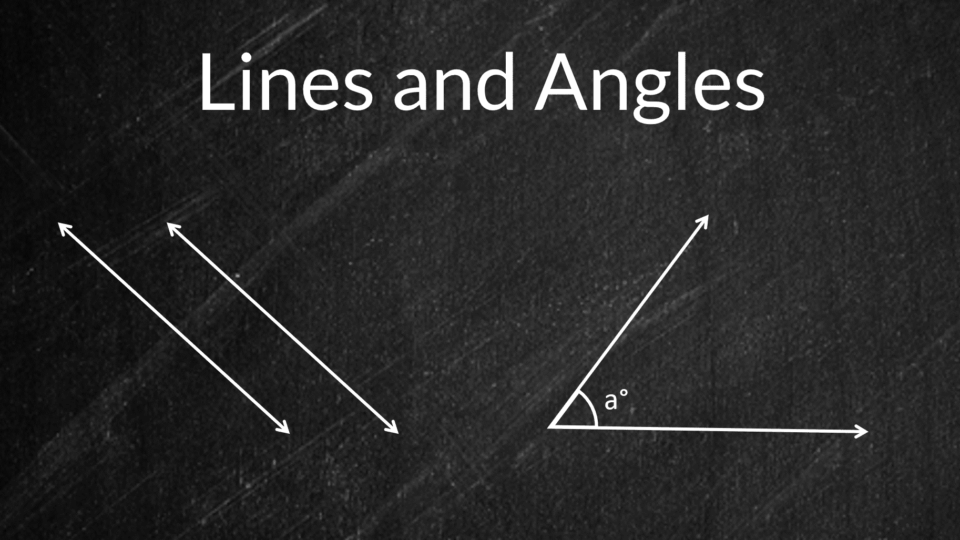# Lines, Angles, And The Properties You Need To Know.

Introduction: In geometry, every figure has some kind of line or angle within them. The lines have a way of intersecting that leads to the formation of an angle. If they don’t meet then you can call them parallel. Such lines never meet or touch each other. However, if you draw a line across both of them, you will find many angles. Each set of acute angles have different properties that you need to know. In your exam, the teacher will not provide the values of every length and angle. Read this article to know how you can use those hints and get the other angles.

Measuring them: In usual cases, you will have a protractor to measure the value of these angles. Even if you don’t, there are other ways of knowing it. An angle is basically how much you have to rotate a line to intersect at a plane. The types of angles a figure has determined what name you give it. If the four angles are 90 degrees then Mathematicians term it as a square. Along with the angle, the length of the sides also gets affected. That is why the square has four sides that have the same measurements.

Contents

## Some common types of angles:

You can find out the type of angle if you know how the two lines meet. Here are the basic categories that students need to learn in Geometry:

Acute angle: The angles have a range within which you can measure them. It starts from zero degrees and goes up to 360. Between them, they have their decimal parts as well. You can refer to an angle as acute if it lies between zero and ninety degrees.

Read More:   Why Inboard Education Is So Important

Obtuse angle: This is like an opposite part of the acute angle. It lies in a range between 180 and 90 degrees. That means that this angle will always be bigger than the acute angle. Every acute angle has an obtuse angle lying next to it. To find it, you just have to subtract it from 180 degrees.

Right and straight: You don’t have to get confused when you hear the word, ‘right angle’. It means the angle that you have measured is just 90 degrees. When the angle is 180 degrees it is known as the straight angle. It is because both the lines are at an angle where they are completely straight to each other.

Reflex: In some cases, the angles may also be greater than 180 degrees. You can call them reflex angles as well. But you need to be sure that it is not equal to 360 degrees. In those situations, Mathematicians explain that a full rotation has been achieved.

### Common properties that angels have:

A majority of angles consist of the same vertex of origination. These are referred to as adjacent and they originate the same way. There is another term that kids will deal with is called complementary. When two angles “a” and “b” give a sum of 90 degrees, they fall in this category. It is an interesting attribute that you can apply to calculate adjacent angles. Knowing the fact that complementary angles are always adjacent is extremely helpful. But that is not the case in angles that are supplementary(total sum is 180 degrees). Even if they are not adjacent you can include them in this category.

Read More:   How GogoPDF Can Fix A Student’s Damaged Files

Knowing the sum of a set of angles is an important feature that students need to apply. For more knowledge, you can use Cuemath, an online platform for learning math and coding by expert teachers.